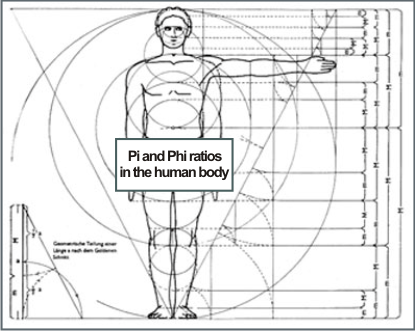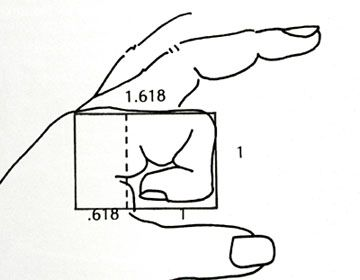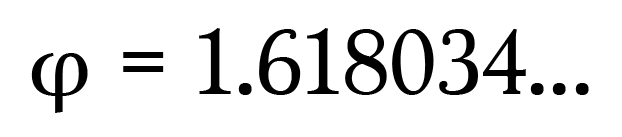## It's all in the Numbers.

"As within, so without." The universe is mathematical formulas, geometric shapes and numeric symbols: The work of a Superior Mastermind. Out of naught came ought, God thought.

0.  Nihil.

1.   Unity.

2.  Duality.

3.  Trinity.

4.  Quaternity.Heptad. (Greek) from heptas. Groups of seven abound; seven days in a week, seven colors in the visible spectrum, seven notes in the music scale, seven chakras, seven continents, seven wonders of the world, seven days of creation: Seven is the Sabbath Day, the number of completion.Numbers also represent notes on the music scale: The language of the universe is sound:  Music!___________________________________________________________________________________

## Sacred Geometry.

"Irrational"  numbers are infinite and transcendental.####Pi is the Greek symbol used to represent a constant: The ratio of the circumference of a circle to its diameter, approximately 3.14159. Pi has been calculated to over one trillion digits beyond its decimal point!Phi Ratio. "The divine proportion." Greek mathematicians first studied what we now call the golden ratio because of its frequent appearance in geometry. The phi ratio has held a special fascination for at least 2,400 years.The Fibonacci Sequence: A series of numbers: 0, 1, 1, 2, 3, 5, 8, 13, 21, 34, ... The next number, or the sum is found by adding up the two numbers before it. The phi ratio is the quotient you get by dividing a number of the Fibonacci sequence by its previous one, = 1.6180...

####In geometry, golden rectangle is a rectangle whose side lengths are in the golden ratio. The Golden Rectangle, according to the phi ratio, is used in architecture since the ancient Greeks. The golden spiral is a logarithmic spiral whose growth factor is φ, the golden ratio: That is it gets wider (or further from its origin) by a factor of φ for every quarter turn it makes. A pattern seen occurring throughout the universe, from the shape of galaxies and hurricanes to a shell picked up on the beach.

#####The following animated piece by Disney artists is a rare treasure.

28:08

________________________________________________________________________________

## The Mandelbrot Set.

set of complex numbers which remains bounded in absolute value; a field first investigated by the French mathematicians Pierre Fatou and Gaston Julia at the beginning of the 20th century. This fractal was first defined and drawn in 1978 by Robert W. Brooks and Peter Matelski as part of a study of Kleinian groups. On 1 March 1980, at IBM's Thomas J. Watson Research Center in Yorktown Heights, New YorkBenoit Mandelbrot (1924-2010), first saw a visualization of the set. Mandelbrot set images may be created by testing the complex numbers for each sample point c,into infinity.##### Zoom sequence of the Mandelbrot set.

The Mandelbrot set has become popular outside mathematics both for its aesthetic appeal and as an example of a complex structure arising from the application of simple rules. It is one of the best-known examples of mathematical visualization. Treating the real and imaginary parts ofas image coordinates on the complex plane, pixels may then be colored.

6:31

Benoit Mandelbrot studied the parameter space of quadratic polynomials in an article that appeared in 1980. The mathematical study of the Mandelbrot set really began with work by the mathematicians Adrien Douady and John H. Hubbard, who established many of its fundamental properties and named the set in honor of Mandelbrot.

The mathematicians Heinz-Otto Peitgen and Peter Richter became well known for promoting the set with photographs. The cover article of the August 1985 Scientific American introduced a wide audience to the algorithm for computing the Mandelbrot set with an image. The Mandelbrot set became prominent in the mid-1980's as a computer graphics demo, when personal computers became powerful enough to plot and display the set in high resolution. There are many programs used to generate the Mandelbrot set and other fractals, some of which are described in fractal-generating software. These programs use a variety of algorithms to determine the color of individual pixels and achieve efficient computation.

1:42

0.53Mandelbox "Alien Hive"

1:45Mandelbulb 3D - The Depths of Europa

3:01